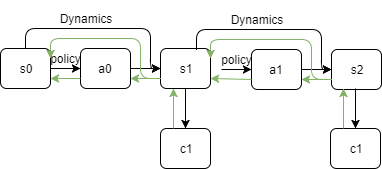# Should we set `requires_grad=False` for this RL computational graph?Suppose we have our computational graph like the figure above (second `c1` should be `c2`), should we actually set `dynamics.parameters.requires_grad=False` and `policy.parameters.requires_grad=False` ?

Since dJ/dpolicy = dc1\dpolicy + dc2\dpolicy = dc1\ds1ds1\ddynamicsddynamics/da0da0/dpolicy + dc2/ds2ds2/ddynamics*[ddynamics/ds1 + ddynamics/da1]
= dc1\ds1ds1\ddynamicsddynamics/da0da0/dpolicy + dc2/ds2ds2/ddynamicsddynamics/ds1ds1/da0da0/dpolicy + dc2/ds2ds2/ddynamicsddynamics/da1da1/dpolicydpolicy/ds1ds1\ddynamicsddynamics/da0da0/dpolicy

So I guess I just the upward gradients from each cost along through the first action a0, According to the formula above, the gradients of policy shouldn’t be accumulated until it reaches the first action, and gradients of dynamics model should not accumulated at all, just let the local-gradients flow from upward to downward (i.e. without += dynamics.parameters.grad)

What is the difference with any classical RL approach? what are c1 and c2?

If you need a simple example for RL, you should look at this: http://pytorch.org/tutorials/intermediate/reinforcement_q_learning.html and this: https://github.com/pytorch/examples/blob/master/reinforcement_learning/reinforce.py

You should use `requires_grad = True` for a variable V only if you want to derivate the operations applied to V: F(V|P) with respect to the parameters P of the operation.

@alexis-jacq
c1 and c2 are cost values of a state. This is actually a direct policy search method. The goal is to optimize the policy which can reduce the summation of costs at each time step. It seems to me that the dynamics model shouldn’t accumulate gradient with (`requires_grad = False`) just not to keep adding up `dynamics.parameters.grad` which might either explodes or provide the wrong gradient to policy network ?

However, in this way, the natural solution should be even the policy network should not accumulate gradient for each time step, only store the gradients to `policy.parameters.grad` when it reaches the first action `a0`. This makes me a bit confused since we can only either set `requires_grad = False/True` to policy network in one time, I don’t know how to set `False` for all other time step and `True` only for first step in `a0` , where the same network is used.

So if you want to optimize your policy (let call it P) with gradient descent, you should have variables taken as input (with is a state S) that requires a grad.

So, if in your code, you have something like this:

``````A = P(S1) # A is the action
S2, C = Dynamic(S1, A) # S' the new state, C the cost function you want to minimize
C.backward()
optimizer.step() # optimizer that updates P in order to optimize C
``````

Then S1 should require a gradient, otherwise, the backward function applied to C will not know what to compute. If you don’t want to accumulate gradient (which I understand) just call `optimizer.zero_grad()` at the beginning of the gradient descent loop.

@alexis-jacq
I think what was described above is

``````Dynamics.requires_grad = False

S0 = leaf Variable
A0 = P(S0)
S1  = Dynamics(S0, A0)
C1 = cost(S1)
A1 = P(S1)
S2 = Dynamics(S1, A1)
C2 = cost(C2)

C1.backward()
C2.backward()

Update parameter of P
``````

This may not be the right way, I guess the topic means during `backward()`, the `P.grad` only stored in `P(S0)`, and when executing `P(S1)` the gradients just flows through

i.e. for `P(S1): requires_grad = False` and `P(S0): requires_grad = True`

This would not work.

In order to run a gradient descent, you need to compute a gradient. So you need to have S0 requiring a gradient, same for S1 and all the states that will be input to your policy network.

Also, it’s cleaner to zero_grad the optimizer at the beginning of the loop in order to avoid “zero-ing” the gradient you are computing.

But before all, are you doing temporal-difference - based learning? In that case you don’t update from C, but from the temporal difference error based on cost C:

``````optim.zero_grad()
values = P(S)
A = greedy_choice(values) # that can be epsilon greedy or softmax
newS = Dynamics(S,A)
newC = Cost(newS)
TD = LossFunction(values, newC+P(newS))
TD.backward()
optim.step()
S = newS
``````

@alexis-jacq
Aha, I see what you mean, yes each of the state `S0/S1` should require gradient. What it was describing is that the parameters of the policy network should not require grads in S1, but need to require grads in S0. Because if we set all parameters requiring gradient, the `parameters.grad` will of a summation of twice. Think of 20 time step, when we backprop with `c20.backward()`, the policy network parameters will added up 20 times gradients.

It is not the case with TD learning, the summation of cost values at each time step is the objective function to backprop w.r.t the parameters of policy network. And the policy network produces continuous action directly.

Not if you call `optimizer.zero_grad()`